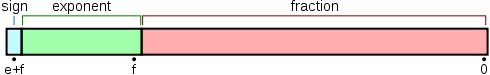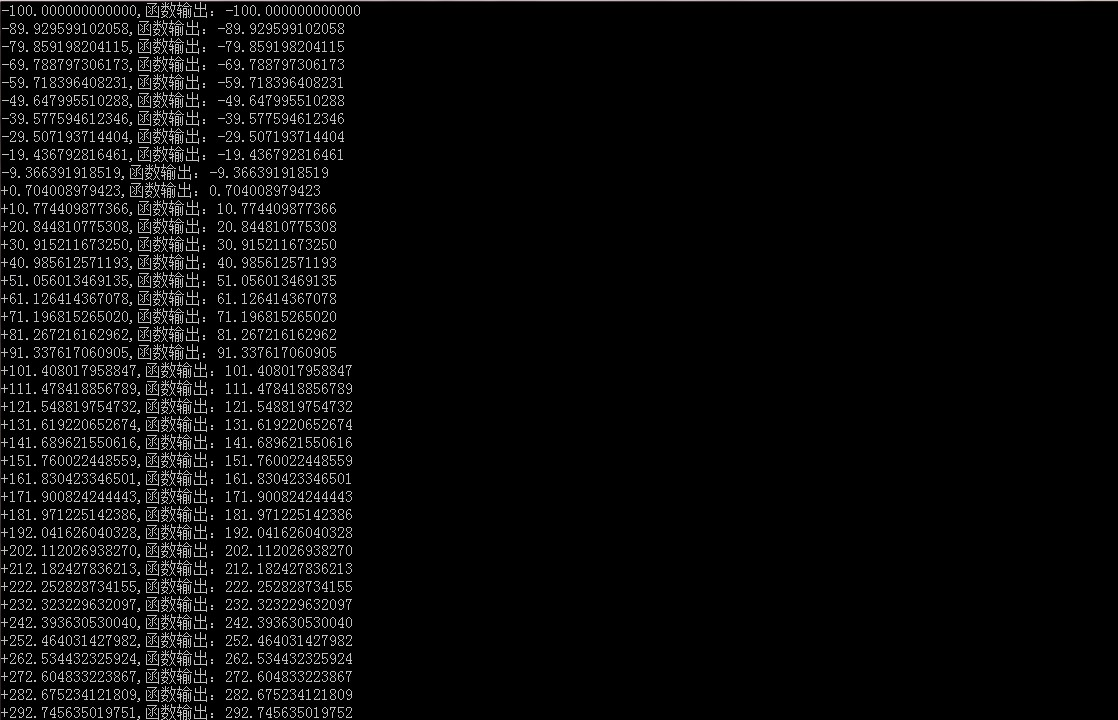• layout: post ...tags: c++ 浮点数起因浮点数的精度IEEE754表示测试浮点数运算浮点数精度我的理解自己的理解总结参考 起因 今天遇到一个问题，角色卡在一个模型边上，在PVD看模型也比较正常。最...
文章目录起因浮点数的精度IEEE754表示测试浮点数运算浮点数精度我的理解自己的理解总结参考
起因
今天遇到一个问题，角色卡在一个模型边上，在PVD看模型也比较正常。最终原因呢是因为模型的一个三角形的两个顶点非常进，结果在浮点数运算的时候这种非常小的差异就被丢掉了，所以在PhysX中会判定移动了距离为0的位置，所以一直就卡在原地，跳也跳不起来。来看下两个顶点的信息：
	 = {x = -4.10000086, y = -0.200000167, z = -3.56512594}
 = {x = -4.10000086, y = -0.200000077, z = -3.56512594}

只有y值有一丁点的差异，这个时候起码还在浮点数的有效范围内。带上几个问题对研究这个问题会有帮助：

1.为什么 -0.200000167和 -0.200000077浮点数表示不一样，最后加上4.76143503(运算用到的一个坐标)结果就一样了？是什么原因？
2.浮点数运算的逻辑是什么？
3.浮点数的精度为什么是7位？

浮点数的精度
IEEE754表示测试
把上面出问题的数据抽出来测试以下
	float y = 4.76143503;
float y1 = -0.200000167;
float y2 = -0.200000077;

float y1Add = y + y1;
float y2Add = y + y2;

std::cout << std::bitset<32>(*(_ULonglong*)&y) << std::endl;
std::cout << std::bitset<32>(*(_ULonglong*)&y1) << std::endl;

std::cout << std::bitset<32>(*(_ULonglong*)&y2) << std::endl;

cout << "fTest = " << y1Add << endl;
cout << "fTest1 = " << y2Add << endl;

结果可以看到：
	01000000100110000101110110101101
10111110010011001100110011011000
01000000100100011111011101000110
10111110010011001100110011010010
01000000100100011111011101000110
fTest = 4.56143
fTest1 = 4.56143

小结：

y1和y2的浮点数表示的确不一样，即IEEE754的23位小数位是满足条件的
做了加法之后，结果变成一样了

那么接下来根据问题来分析原因是什么样的。
浮点数运算
参考，的主要步骤：

1.规格化表示
2.对阶
3.尾数标数
4.规格化
5.舍入

	IEEE 754 standard floating point Addition Algorithm
Floating-point addition is more complex than multiplication, brief overview of floating point addition algorithm have been explained below
X3 = X1 + X2
X3 = (M1 x 2E1) +/- (M2 x 2E2)
1) X1 and X2 can only be added if the exponents are the same i.e E1=E2.
2) We assume that X1 has the larger absolute value of the 2 numbers. Absolute value of of X1 should be greater than absolute value of X2, else swap the values such that Abs(X1) is greater than Abs(X2).
Abs(X1) > Abs(X2).
3) Initial value of the exponent should be the larger of the 2 numbers, since we know exponent of X1 will be bigger , hence Initial exponent result E3 = E1.
4) Calculate the exponent's difference i.e. Exp_diff = (E1-E2).
5) Left shift the decimal point of mantissa (M2) by the exponent difference. Now the exponents of both X1 and X2 are same.
6) Compute the sum/difference of the mantissas depending on the sign bit S1 and S2.
If signs of X1 and X2 are equal (S1 == S2) then add the mantissas
If signs of X1 and X2 are not equal (S1 != S2) then subtract the mantissas
7) Normalize the resultant mantissa (M3) if needed. (1.m3 format) and the initial exponent result E3=E1 needs to be adjusted according to the normalization of mantissa.
8) If any of the operands is infinity or if (E3>Emax) , overflow has occurred ,the output should be set to infinity. If(E3 < Emin) then it's a underflow and the output should be set to zero.
9) Nan's are not supported.

然后，我就自己手动计算了一下：
	01000000100110000101110110101101 = 4.76143503
阶码：10000001 = 129 - 127 = 2
尾数：1.00110000101110110101101

--------------------------------------------------
10111110010011001100110011011000 = -0.200000167
阶码：01111100 = 124 - 127 = -3
尾数：1.10011001100110011011000

01000000100100011111011101000110 = 4.56143475 = 4.76143503 + -0.200000167
阶码：10000001 = 129 - 127 = 2
尾数：1.00100011111011101000110

加法运算：
1.1001 1001 1001 1001 1011 000
对齐：小数点左移2-(-3) = 5位
0.00001.1001 1001 1001 1001 1011 000
相减：
1.0011 0000 1011 1011 0101 101
-	1.0000 1100 1100 1100 1100 110  11 000
= 	1.0010 0011 1110 1110 1000 111
比较	1.0010 0011 1110 1110 1000 110

--------------------------------------------------
10111110010011001100110011010010 = -0.200000077
阶码：01111100 = 124 - 127 = -3
尾数：1.10011001100110011010010

01000000100100011111011101000110 = 4.56143475 = 4.76143503 + -0.200000077
阶码：10000001 = 129 - 127 = 2
尾数：1.00100011111011101000110

加法运算：
1.10011001100110011010010
对齐：小数点左移2-(-3) = 5位
0.0000110011001100110011010010
相减：计算器去掉小数点和前面的1来的比较快
1.0011 0000 1011 1011 0101 101
-	0.0000 1100 1100 1100 1100 110	10010
=	1.0010 0011 1110 1110 1000 111
比较	1.0010 0011 1110 1110 1000 110


小结：

计算的结果的确是一样的，但是和最终的4.76143503还是有点不一样（最后一个比特位），这说明我手动计算和机器计算还是有点区别，包括后面的规格化，舍入我就没去细看了，留个问题在这里吧

	// 4.76143503 + -0.200000167
1.0010 0011 1110 1110 1000 111
// 4.76143503 + -0.200000077
1.0010 0011 1110 1110 1000 111
// 最终结果的二进制
1.0010 0011 1110 1110 1000 110


这里解释了前两个问题：最主要是在浮点数的运算过程中(这里是加法)，因为要对齐阶码，所以更小的数字的尾数后面几位（这里是5位）都忽略掉了，所以-0.200000167和 -0.200000077的差异也就没有了

再来看看IEEE754有23位小数，为什么精度是7位小数位？
浮点数精度
先确定下自己的问题：

精度指的是十进制的小数点后面的个数，而IEEE754标准的23位指的是二进制有效位
我想理解的是23位二进制有效位是如何换算到十进制的7位有效位的
网上搜索了之后，最终可以确定的是十进制小数点后面可以精确到6-7位
自己最终问题是如何用数学方法证明?

最常见的解释如下，参考：

因为float类型的数值由二进制下的后23位决定的，而这后23位表示的十进制的数最大为2^23=8388608，也就是说在二进制下能表示的准确的23位的数转换 到十进制下最大的数是7位的，数值是多少不重要，因为这个数是在十进制下是7位，所以float在十进制下的精度位7位。再说白一点，二进制下能表示的最大的准确的数值转换为十进制是7位。

简单来讲，就是二进制可以表示的8388608是一个7位数字，但是并不能包括完全包括所有7位有效位，所以是6-7位有效位。但是，这里我还是有疑问的，这个算法应该是小数点前面可以这么算，小数点后面怎么算的，如果可以这么算，又如何解释？
我的理解
参考之后，相对而言，我比较喜欢的解释。但最终我自己理解又是另外一个。

1.小数点后面的二进制和十进制转换应该是这样的表达式，当然应该要乘以一个0或者1表示有效位上面有数据

$0.xxxx = 2^{-1} + 2^{-2} + ... + 2^{-23}$
那么，小数点后面最小的单位是$2^{-23} =$
这里其实也说明了浮点数的有些数值只能是近似表示的

2.数学推导

$-{log_{10}}{2^{-23}} = 6.924$
自己的理解

(1)23位小数，能表示的最小小数位，其他的小数都是乘以这个最小单位构成的

$2^{-23}= 0.00000011920928955078125$
一开始我想，这后面明显有这么多小数，为什么是7位？

(2)这个最小单位表示的就是精度的尾数，怎么理解！
如果最小单位是0.1，那么精度就是小数点后面1位，因为你永远也组合不了0.1后面的小数(整数个最小单位求和)，比如0.01、0.02等
如果最小单位是0.000001，那么精度就是小数点后面6位，同样的你也永远组合不了0.000001更小单位的小数，比如0.0000001、0.0000002等等
(3)准确来说二进制23位有效位表示的精度为小数点后面6~7位。
由上面一条可以知道，0.00000011920928955078125这个最小单位用23位二进制任意组合(求最小单位的倍数)，只能得到比这个0.00000011920928955078125更大的数。所以永远得不到0.00000001这样的小数（精度为小数点后面8位），但是肯定可以得到0.000001（精度为小数点后面6位）的小数。但是不能完全表示精度位小数点后面为7位的小数。下面忽略舍入的算法，只取小数点后面7位有效位的计算结果：

0.00000011920928955078125 * 1 = 0.0000001
0.00000011920928955078125 * 2 = 0.0000002
0.00000011920928955078125 * 3 = 0.0000003
0.00000011920928955078125 * 4 = 0.0000004
0.00000011920928955078125 * 5 = 0.0000005
0.00000011920928955078125 * 6 = 0.0000007
0.00000011920928955078125 * 7 = 0.0000008
0.00000011920928955078125 * 8 = 0.0000009
0.00000011920928955078125 * 9 = 0.000001

可以看到0.0000006表示不出来，如果考虑舍入的话可能是其他的表示不出来，所以说不能完全表示所有的小数点后面7位小数
总结

浮点数的爱与恨

参考
深入理解浮点数有效位
二进制浮点数的加减法运算
Floating Point Tutorial
程序员必知之浮点数运算原理详解
float的精度为什么是7位详解
java浮点类型float和double的主要区别，它们的小数精度范围大小是多少？
浮点计算精度损失原因
单精度浮点数的有效数字为什么是7位，我算的明明是6位，你看我算的对吗？
Why IEEE754 single-precision float has only 7 digit precision?
In-depth: IEEE 754 Multiplication And Addition
浮点数精度问题透析:小数计算不准确+浮点数精度丢失根源
关于float型是单精度的有效位数是7位,为什么在下面的例子中这8位都是准确的呢？
二进制计算器网页版


展开全文c++ 浮点数
• 故特意查找了一下单精度、双精度小数的二进制编码，自己实现一个输出十进制字符串的函数。具体代码如下所示。 #include"decimalString.h" #include int lengthOfInt(int a);//a十进制绝对值的位数 //整数a十进制...
在C语言里，用printf即可格式化输出小数，但是在某些特殊的场合，这些库函数却是不能使用的。故特意查找了一下单精度、双精度小数的二进制编码，自己实现一个输出十进制字符串的函数。具体代码如下所示。
#include"decimalString.h"
#include<stdio.h>

//若judge为0则返回ret值
#define RETURN_IF_ZERO(judge,ret) do{if(!(judge))return (ret);}while(0)#define MARK(len) ((1<<(len))-1)//二进制长度len的掩码
#define LOW_BINARY(d,low) ((d)&MARK(low))//取双字d的低low位
#define HIGH_BINARY(d,high) (((d)>>(32-(high)))&MARK(high))//取双字d的高high位
int lengthOfInt(int a);//a十进制绝对值的位数
//整数a十进制写入到字符串buf中
char*intToStr(int a,char*buf,int*length);
//将小数点后若干高位写入字符串，高位为0需补上
//0<=decimal<1,且写入位数writeLen<=7
char*decimalToStr(double decimal,int writeLen,char*buf);

int floatToInt(float f){//单精度的整数部分
return doubleToInt(f);
}
//双精度小数取整
//适用范围：数值在int整型范围以内
int doubleToInt(double db){
const int eOffset=1023;//指数偏移
const int wWidth=52,eWidth=11;//尾数位数，指数位数
int *binary=(int*)&db;
int sign,e,w,r;//e:指数，w:尾数
sign=binary>=0?1:-1;//正负号
e=LOW_BINARY(HIGH_BINARY(binary,1+eWidth),eWidth);
e-=eOffset;
RETURN_IF_ZERO(e>=0,0);
RETURN_IF_ZERO(e!=0,sign);
RETURN_IF_ZERO(e<=30,0x7ffffff*sign);
w=LOW_BINARY(binary,wWidth-32);
w<<=(64-wWidth);
w|=HIGH_BINARY(binary,64-wWidth);
r=(1<<e)|HIGH_BINARY(w,e);
return sign*r;
}
//双精度小数f转换成小数位数precision位(最多13位小数)的十进制字符串
//小数范围需要在int范围以内
char*doubleToStr(double f,int precision,char*buf){
const int limit=7,dLimitTime=10000000;//小数位数一次写入位数最大限制
int len,zPart,dHign,sign;
char*tmp=buf;
double js=0.5;//四舍五入近似
sign=f<0?-1:1;//正负号
if(sign==-1)
*tmp++='-';
f*=sign;//取绝对值
precision=precision<0?0:precision;
precision=precision>13?13:precision;
len=precision;
while(len-->0)
js/=10;
f+=js;//四舍五入
zPart=doubleToInt(f);//整数部分
intToStr(zPart,tmp,&len);//写入整数部分
if(precision>0){//小数位数要求>0
dHign=precision<=limit?precision:limit;
tmp[len]='.';
tmp+=len+1;
f=f-zPart;//小数部分
decimalToStr(f,dHign,tmp);//小数高位部分先写入字符串
if(precision>limit){//limit位之后的小数的需要另外写入
f=f*dLimitTime;//放大倍数
f-=doubleToInt(f);//
decimalToStr(f,precision-limit,tmp+limit);
}
}
return buf;
}
//单精度小数f转换成小数位数precision位(最多7位小数)的十进制字符串
//小数范围需要在int范围以内
char*floatToStr(float f,int precision,char*buf){
precision=precision>7?7:precision;
return doubleToStr(f,precision,buf);
}
//整数a十进制写入到字符串buf中
char*intToStr(int a,char*buf,int*length){
int pos=0;
char*tmp;
if(a<0){
a*=-1;
buf[pos++]='-';
}
tmp=buf+pos+lengthOfInt(a);
if(length!=NULL)*length=tmp-buf;
*tmp='\0';
while(a>9){
*--tmp=0x30|(a%10);
a/=10;
}
*--tmp=0x30|a;//0x30='0'
return buf;
}
//将小数点后若干高位写入字符串，高位为0需补上
//0<=decimal<1,且写入位数writeLen<=7
char*decimalToStr(double decimal,int writeLen,char*buf){
int t,tmp=writeLen;
t=1;
while(tmp-->0)
t*=10;
tmp=doubleToInt(decimal*t);//乘上10^writeLen再取整
buf[writeLen]='\0';
for(t=--writeLen;t>=0;t--){
buf[t]=(tmp%10)|0x30;
tmp/=10;
}
return buf;
}
int lengthOfInt(int a){//a十进制绝对值的位数
int len=1;
a=a>0?a:-a;
while(a>9){
len++;
a/=10;
}
return len;
}

测试函数如下：
void testFDouble(){
double d2=12;
float f2=-100,f3=12.8,f4=0.09;
char tmp;
for(int k=0;k<40;k++){
double f=f2+k*10.0704008979423463423;
//printf("%f-强制转换整数：%d\n",f,doubleToInt(f));
printf("%+.12f,函数输出：%s\n",f,doubleToStr(f,12,tmp));
}
//printf("%f-强制转换整数：%d\n",-0.1,doubleToInt(-0.1));
//printf("%f-强制转换整数：%d\n",0.1,doubleToInt(0.1));
//printf("%f-强制转换整型：%d；函数转换：%d\n",f3,(int)f3,floatToInt(f3));
//printf("%f-强制转换整型：%d；函数转换：%d\n",f4,(int)f4,floatToInt(f4));
//printf("%s\n",intToBinary(12,tmp));
//printf("%s\n",intToBinary(*(int*)&f2,tmp));
//printf("%s\n",doubleToBinary(d2,tmp));
}

结果如下：展开全文c语言
• C# 四个字节十六进制单精度浮点数之间的相互转化 即是所谓的IEEE754标准,这也是大多数硬件存储浮点数的标准。单精度浮点数占4个字节，表示范围：在负数的时候是从 -3.402823E38 到 -1.401298E-45，而在正数的...
C# 四个字节十六进制数和单精度浮点数之间的相互转化
即是所谓的IEEE754标准,这也是大多数硬件存储浮点数的标准。单精度浮点数占4个字节，表示范围为：在负数的时候是从 -3.402823E38 到 -1.401298E-45，而在正数的时候是从 1.401298E-45 到 3.402823E38 。
一、在C#中的转换函数为：
1,由四个字节的十六机制数组转浮点数：
    byte[] bytes = new byte;

BitConverter.ToSingle(bytes, 0);//从第0个字节开始转换

2,由浮点数转数组：
byte[] bytes = BitConverter.GetBytes(floatValue);

1》这种转换方法经常用于串口通讯中，表示范围足够各种传感器数值传输及工控场合，将要发送的浮点数据转换为4个字节的十六机制数，然后由串口发出，在接收端再将其转换为浮点数。
2》单片机或非.net环境下使用转换程序则不能调用BitConverter类！
二、提供以下代码以供转换：
1》未修改过的如下：可以在C#中直接调用而不用库函数
public static float ToFloat(byte[] data)
{
float a = 0;
byte i;
byte[] x = data;
unsafe
{
void* pf;
fixed (byte* px = x)
{
pf = &a;
for (i = 0; i < data.Length; i++)
{
*((byte*)pf + i) = *(px + i);
}
}
}

return a;
}

public static byte[] ToByte(float data)
{
unsafe
{
byte* pdata = (byte*)&data;
byte[] byteArray = new byte[sizeof(float)];
for (int i = 0; i < sizeof(float); ++i)
byteArray[i] = *pdata++;

return byteArray;
}
}

2》如果对工程进行直接编译会报出一下错误：这是因为C#默认不提供指针支持，只有在不安全代码的形式下才可以用指针。
错误 1 不安全代码只会在使用 /unsafe 编译的情况下出现 E:\Visual Studio 2008\Projects\TEST\testOfFloatConsolt\testOfFloatConsolt\Program.cs 26 13 testOfFloatConsolt
这时选择VS的菜单栏中的项目->"Project"属性->生成->常规->允许不安全代码 勾选即可

三、单片机串口通讯浮点转换函数
我在AVR串口通信协议中用到了这部分，直接将单片机的运算结果（浮点类型）转换为（字节类型）嵌入串口通信协议中，上传至上位机。
下面为符合IEEE754标准将浮点数转换为四个字节的数组的函数源代码：已经用于mega16单片机的串口通信中。
WinAVR-20090313测试通过：
void FloatToByte(float floatNum,unsigned char* byteArry)
{
char* pchar=(char*)&floatNum;
for(int i=0;i<sizeof(float);i++)
{
*byteArry=*pchar;
pchar++;
byteArry++;
}
}

四、下面为符合IEEE754标准的由四个字节型数组转化为相应的浮点数
WinAVR-20090313测试通过：
float ByteToFloat(unsigned char* byteArry)
{
return *((float*)byteArry);
}

调用测试方法：其中USART_Transmit();为向串口发送的函数。
unsigned char floatToByte;
FloatToByte(12.15,floatToByte);
float a=ByteToFloat(floatToByte);
FloatToByte(a,floatToByte);
USART_Transmit(floatToByte);
USART_Transmit(floatToByte);
USART_Transmit(floatToByte);
USART_Transmit(floatToByte);

//在上位机用串口进行读取时调用

BitConverter.ToSingle(bytes, 0);

//就会转换成12.15，测试方法可以随着需求改变。



展开全文• 原文地址：用四个字节十六进制表示单精度浮点数作者：无名指 即是所谓的IEEE754标准,这也是大多数硬件存储浮点数的标准。单精度浮点数占4个字节，表示范围：在负数的时候是从 -3.402823E38 到 -1.401298E-45，...
原文地址：用四个字节十六进制数表示单精度浮点数作者：无名指

即是所谓的IEEE754标准,这也是大多数硬件存储浮点数的标准。单精度浮点数占4个字节，表示范围为：在负数的时候是从 -3.402823E38 到 -1.401298E-45，而在正数的时候是从 1.401298E-45 到 3.402823E38 。

在C#中的转换函数为：

1,由四个字节的十六机制数组转浮点数：

byte[] bytes = new byte;

BitConverter.ToSingle(bytes, 0);

2,由浮点数转数组：

byte[] bytes = BitConverter.GetBytes(floatValue);

这种转换方法经常用于串口通讯中，表示范围足够各种传感器数值传输及工控场合，将要发送的浮点数据转换为4个字节的十六机制数，然后由串口发出，在接收端再将其转换为浮点数。

单片机或非.net环境下使用转换程序则不能调用BitConverter类！

提供以下代码以供转换：

未修改过的如下：可以在C#中直接调用而不用库函数

public static float ToFloat(byte[] data)
{
float a = 0;
byte i;
byte[] x = data;
unsafe
{
void* pf;
fixed (byte* px = x)
{
pf = &a;
for (i = 0; i < data.Length; i++)
{
*((byte*)pf + i) = *(px + i);
}
}
}

展开全文• 在Access中单精度类型在运算过程中会有产生精度误差，比如，一个双精度乘于一个单精度的值四舍五入保留两位小数的双精度会有精度问题。   求KCMJ: KCMJ=TBMJ*KCXS，并将KCMJ四舍五入保留两位小数。 其中...
• 这种结构是一种科学计数法，用符号、指数和尾数来表示，底数定2—— 即把一个浮点数表示尾数乘以2 的指数次方再添上符号。下面是具体的规格：  符号位 阶码 尾数 长度  float 1 8 23
• 关于16进制浮点数对于大小32-bit的浮点数（32-bit为单精度，64-bit浮点数双精度，80-bit扩展精度浮点数），1、其第31 bit符号位，0则表示正数，反之复数，其读值用s表示；2、第30～23 bit，其读...
• 一、单精度浮点数表示 ...2.75转化为单精度浮点数 2. 两个IEEE754格式小数的加减法 若阶码不同，低阶化为高阶 减法： 符号：直接根据十进制式子的结果的正负确定符号位 数值：直接用绝对值大的减绝对值小的 ...
• 摘　要:在近日几个帖子里面，和QQ群的讨论里面，我发现很多网友都遇到的问题都是因为不恰当地使用了单精度/双精度数值。因此想专门就这个话题谈一下。 正　文: 前言 　单精度和双精度数值类型最早出现在C语言中...存储 integer 语言 数据库 vba
• 单精度浮点数用4字节（32bit）表示浮点数 ...对于单精度浮点数Float: 当数据范围在±131072（65536×2）以内的时候，float数据精度是正确的，但是超出这个范围的数据就不稳定，没有发现有相关的参数设float mysql oracle 数据库
• 参考深入理解C语言-03-有符号，定点，浮点数 1.1定点 一般在没有FPU寄存器的嵌入式系统中使用比较多。比如常见的32位系统中，将高16位作为整数部分，低16位作为小数部分。 这样就可以用整数来模拟定点的.....
• 前提：以下都是建立在IEEE754标准中 2^8 = 256 2^7 = 128 带符号8位2进制本来应该是[-127, -0]U[+0, 127] 而该标准规定： E = 0000 0000 M = 000…000 用来表示了正、负零 E = 1111 1111 M = 000…000 用来表示了正...
• SSE（Streaming SIMD Extensions）指令是一种SIMD 指令， Intrinsics函数则是对SSE指令的函数封装，利用C语言形式来调用...关于本文实现了单精度浮点数组的求和，切实感受SSE带来的速度提升。本文代码主要来自. 首SSE 程序优化
• 首先我们知道单精度型占4个字节的大小也就是32个bit位和整形大小一样 但是存储方式却有很大的区别。 32个bit位可以分为三个部分 0 00000000 00000000000000000000000 1 8 23 （位数） 第一部分是 符号部分存储 内存
• 基础知识：十进制转十六进制；十六进制转二进制；...这种结构是一种科学计数法，用符号、指数和尾数来表示，底数定2——即把一个浮点数表示尾数乘以2的指数次方再添上符号。下面是具体的规格： 符号位 阶码存储 float 编译器 c
• 时　间:2007-11-7 10:17:04 作 者:LucasLynn 摘　要:在近日几个帖子里面，和QQ群的讨论里面，我发现很多网友都遇到的问题都是因为不恰当地使用了单精度/双精度数值。因此想专门就这个话题谈一下。...数据库 存储 integer 语言 vba
• num = str(input("输入一个，显示其浮点数在二进制中的存储，输入exit退出：")) if num=="exit":#此处几个if是float定义的几个量 print("退出运算") break if num=="0":#此处几个if是float定义的几个量 ...
• 先进行名词解释reinterpret_cast是C++里的...以下这段话摘自csdn网友 gzmhero因为单精度浮点是32bits，而整型一般也是32bits的。所以直接将地址取出，按想要的格式输出即可实现单精度到整型的转化。 我的代码： float xfloat c
• 最近在搞DSP，所以不可避免地会遇到浮点数，包括半精度浮点(16bit) 和单精度浮点(32bit)。 1、根据wiki百科介绍，IEEE 754规范中规定的32bit float point的格式：（以下引用wiki百科...浮点
• 并编写主函数进行测试，数据类型可以是整型、单精度型、双精度型。 函数模板原型如下： template T Sort( T arry[ ], int n) //其中n为数组元素个 /////////////////////////////////////// 主要的是怎么通过这个...编程语言 面向对象编程
• 以下是该标准对浮点数格式的描述。 [编辑]本文表示比特的约定 把W个比特(bit)的数据，从内存地址低端到高端，以0到W−1编码。通常将内存地址低端的比特写在最右边，称作最低有效位(least significant bit或lsb)，...
• 摘要： 由于计算机是用二进制来存储和处理数字，不能...为了避免产生精度差异，把需要计算的数字升级（乘以10的n次幂）成计算机能够精确识别的整数，等计算完毕再降级（除以10的n次幂），这是大部分编程语言处理精度
• 　本文面对对SSE等SIMD指令集有一定基础的读者，以单精度浮点数组求和例演示了如何跨平台使用SSE、AVX指令集。因使用了stdint、zintrin、ccpuid这三个模块，可以完全避免手工编写汇编代码，具有很高可移植性。支持...gcc windows linux
•  A、运算符两边的运算必须是数字，因此下面的代码是错误的：  \${3*"5"}  B、使用+（既可以作为加号，也可以作为字符串连接运算符）运算时，如果一边是数字，一边是字符串，就会自动将数字转化字符串。...freemarker
• 精度范围之外,多余位将被忽略,从而分不同情况 : 以下是小数位9,8,7的验证.结果证明 7位有效小数位数,>7位的都忽略. 若多余位之前的有效位数字相等 , 则判断相等 , 既多余位已经不起作用; 若多余位...
• 基于混合最优算法的高精度数控直流电源设计[日期：2008-8-6]来源：中电网 作者：陈伟杰 张虹[字体：大 中 小] 1 设计任务设计并制作数控直流电流源。输入交流200～240V，50Hz；输出直流电压≤10V。原理框图如1所示...算法 语言 汇编 优化
• 浮点数（float，double）表范围和精度问题 浮点数（float，double）表范围和精度问题 其实之前就遇到过浮点数精度丢失的问题，但是一直没有去深入研究，只是停留在知识记忆的层面，久而久之发现之前的...浮点数 float double...